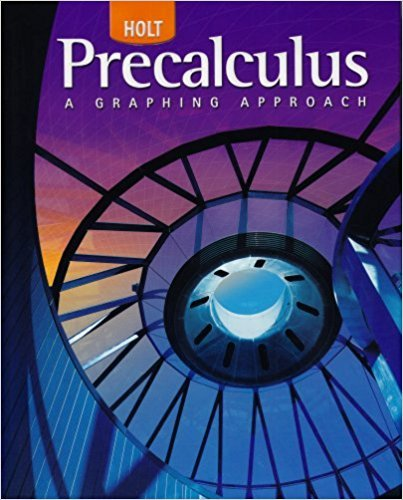×

×

# Solutions for Chapter 3.5: Operations on Functions## Full solutions for Precalculus | 1st Edition

ISBN: 9780030416477Solutions for Chapter 3.5: Operations on Functions

Solutions for Chapter 3.5
4 5 0 239 Reviews
21
0
##### ISBN: 9780030416477

Chapter 3.5: Operations on Functions includes 63 full step-by-step solutions. This textbook survival guide was created for the textbook: Precalculus, edition: 1. This expansive textbook survival guide covers the following chapters and their solutions. Since 63 problems in chapter 3.5: Operations on Functions have been answered, more than 26306 students have viewed full step-by-step solutions from this chapter. Precalculus was written by and is associated to the ISBN: 9780030416477.

Key Calculus Terms and definitions covered in this textbook
• Arrow

The notation PQ denoting the directed line segment with initial point P and terminal point Q.

• Chord of a conic

A line segment with endpoints on the conic

• Circular functions

Trigonometric functions when applied to real numbers are circular functions

• Intercepted arc

Arc of a circle between the initial side and terminal side of a central angle.

• Logarithmic form

An equation written with logarithms instead of exponents

• Minor axis

The perpendicular bisector of the major axis of an ellipse with endpoints on the ellipse.

• nth power of a

The number with n factors of a , where n is the exponent and a is the base.

• nth root

See Principal nth root

• Parametric equations

Equations of the form x = ƒ(t) and y = g(t) for all t in an interval I. The variable t is the parameter and I is the parameter interval.

• Polar coordinate system

A coordinate system whose ordered pair is based on the directed distance from a central point (the pole) and the angle measured from a ray from the pole (the polar axis)

• Positive linear correlation

See Linear correlation.

• Quartic regression

A procedure for fitting a quartic function to a set of data.

• Rational zeros theorem

A procedure for finding the possible rational zeros of a polynomial.

• Scientific notation

A positive number written as c x 10m, where 1 ? c < 10 and m is an integer.

• Solve an equation or inequality

To find all solutions of the equation or inequality

• Standard form of a polar equation of a conic

r = ke 1 e cos ? or r = ke 1 e sin ? ,

• Stretch of factor c

A transformation of a graph obtained by multiplying all the x-coordinates (horizontal stretch) by the constant 1/c, or all of the y-coordinates (vertical stretch) of the points by a constant c, c, > 1.

• Sum of an infinite series

See Convergence of a series

• Unit ratio

See Conversion factor.

• Vertical line

x = a.